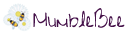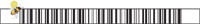# Free Amazon Android App of the Day 9/19/2014

Free Amazon Android App of the day for 9/19/2014 only!

Normally \$1.99 but for today it is FREE!!

## Product Features

• Matrix Calculator
• Graphing Calculator
• Equation Solver
• Unit Coverter
• Currency Converter

## Product Description

“BisMag Calculator 3D” is a powerful math tool for Android consists of 5 calculators. “Matrix Calculator”, a tool to calculate the decompositions and various operations on matrix, “Equation Solver” an instrument capable of solving equations of degree n, “Graphing Calculator” a real scientific graphing calculator can draw graphs in 2D and 3D, “Currency Converter” a currency converter always updated with the new exchange rates and “Unit Converter” a small units converter. In addition we find a comfortable Periodic Table of Elements.

Features in detail:

– Matrix Calculator:

–Derived Quantities
Determinant
Rank
Inverse
PseudoInverse

–Decompositions
Cholesky decomposition
LU decomposition whit pivoting
QR decomposition
SVD – Singular Values decomposition
Eigenvalues – Eigenvectors

–Linear Systems
Linear Systems M N

– Graphing Calculator:

–Sample expressions

variable evaluation: pi
function evaluation: sin(0)
variable definition: a=3.5
function definition: f(x)=x^2-1
parentheses: (1-x)^2

–Logarithms and power

sqrt(x): square root; x^0.5
cbrt(x): cube root; x^(1/3)
exp(x): exponential; e^x
log(x), ln(x): natural logarithm
log2(x), lb(x): binary logarithm
log10(x), lg(x): decimal logarithm
log(base,x): arbitrary base logarithm

sin(x), cos(x), tan(x)
asin(x), acos(x), atan(x)

–Trigonometric – degrees

sind(x), cosd(x), tand(x)
asind(x), acosd(x), atand(x)

–Hyperbolic

sinh(x), cosh(x), tanh(x)
asinh(x), acosh(x), atanh(x)

–Other

gcd(x,y): greatest common divisor
comb(n,k): combinations
perm(n,k): permutations
min(x,y), max(x,y)
floor(x), ceil(x)
abs(x): absolute value
sign(x): signum
rnd(): random value from [0,1). rnd(max): random value from [0, max).
gamma(x): (x-1)!
mod(x,y): modulo

–Complex numbers

i or j is the complex base. Example:
i*i
(1+i)^2
e^(i*pi)

–Operators

+ – × ÷ basic arithmetic
^ power
% percent
! factorial
# modulo
√ square root
‘ first derivative

Value converter in binary, octal or hexadecimal input prefixing respectively 0b, 0o, 0x, in decimal.
binary: 0b1010
octal: 0o17
hexa: 0x100

–Derivative
It is possible to compute the first derivative of a function with one argument using the prime notation: log'(5).
The prime mark (quote) must appear immediately after the name of the function, and must be followed by open-parentheses.
The derivative may be plotted e.g. sqrt'(x).
To compute the derivative of an expression you must define the expression as a named function.
Eg f (x) = x ^ 2 + x, after insertion, type f ‘(x) to display the graph of the derivative.

–Multi plot

To plot multiple functions on the same 2d graph, simply enter them on the same line separated by “;”.

–Special Function

Indefinite Integrals
Limit of a function /*Still in beta*/
Derivative
Definite Integrals
Taylor Series
Tangent Line
Arc Length & Surface Area
Formula Tables

–Graph (MultiPlot)

Graph of parametric functions
Graph in polar coordinates
MultiPlot 3D

— Widget Calculator

http://www.tuttoandroid.net/applicazioni/app-nursery-bismag-calculator-3d-113391/

http://appszoom.com/android_applications/tools/bismag-calculator-3d_czsgc.html

http://www.androidworld.it/2013/05/25/italia-chiama-android-bismag-calculator-3d-speeder-nasoni-ditalia-159363/#

http://www.androidtapp.com/bismag-calculator-3d/

http://www.androidgeek.it/applicazioni-android/app-world/bigmag-calculator-3d-una-mente-matematica-per-il-tuo-android/

Follow Me On Social Media